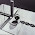## Thursday, July 28, 2016

### Python console progress bar (using \b and \r)

One of the things that really fascinated me in console applications is when I see text being changed on the same line without new lines being added. An example would be a progress bar or some percentage being updated on the same line like this:The idea is to use ASCII control characters which control the console's behaviour. These control characters were most useful back when computer displays were typewriters that printed out the text that the program wanted to show but are now still useful in console applications.

First of all, the code shown below only works in the command prompt / terminal and not in IDLE. To try them out open command prompt (if using Windows) or terminal (if using Linux) and enter the command "python" which will open a stripped down version of IDLE. Alternatively you can write a script file and then call it through the command prompt / terminal by entering the command "python <script file>" such as "python C:\file.py".

Control characters

There are two control characters of interest here:

\b moves the cursor one character back, which will print the following character over the previous character. This is useful for overwriting the characters at the end of the line.
print('xy\bz')\r moves the cursor to the beginning of the line, which will print the following character over the first character. This is useful for overwriting the characters at the beginning of the line or the whole line.
print('xy\rz')So how do we use these to display a functioning progress bar? First of all, if we're using \b then we'll probably need to overwrite a number of characters at the end, not just one. To do this, just print \b as many times as needed:
print('xyyy\b\b\bzzz')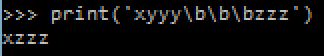Separate prints

We also probably want to use separate prints rather than put everything in the same print statement. To do this you need to make sure that the print will not start a new line at the end. In Python 3 you can add the argument "end=''" to the print:
def f():
print('xy', end='')
print('\bz')

f()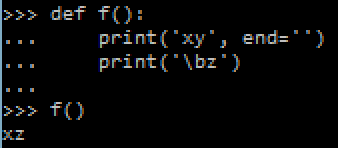def f():
print('xy', end='')
print('\rzz')

f()Alternatively you can use the sys.stdout.write function which is equivalent to print without the extra features such as adding a new line at the end:
import sys

def f():
sys.stdout.write('xy')
sys.stdout.write('\bz\n')

f()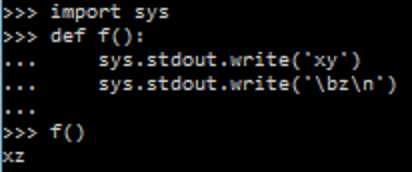String formatting

When displaying a percentage it's important that the number always takes the same amount of character space so that you know how many characters to overwrite. This can be done using the format method of strings. The format method allows you to make a string take a fixed amount of characters, filling the remainder with spaces as follows:
curr = 12
total = 21
frac = curr/total
print('[{:>7.2%}]'.format(frac))The formatting code is the "{:>7.2%}", where ">" means that the string is be right aligned with spaces added to the front, "7" is the fixed length of the string + padded spaces (3 whole number digits + a point + 2 decimal digits + a percentage sign) ".2" is the number of decimal places to round the percentage to, and "%" means that the fraction should be expressed as a percentage.

Replicated strings

When displaying a progress bar it would also be useful to know that you can replicate a string for a number of times using the '*' operator, as follows:
curr = 12
total = 21
full_progbar = 10
filled_progbar = round(curr/total*full_progbar)
print('#'*filled_progbar + '-'*(full_progbar-filled_progbar))Full example

Here is a full progress bar example:

def progbar(curr, total, full_progbar):
frac = curr/total
filled_progbar = round(frac*full_progbar)
print('\r', '#'*filled_progbar + '-'*(full_progbar-filled_progbar), '[{:>7.2%}]'.format(frac), end='')

for i in range(100000+1):
progbar(i, 100000, 20)
print()It might also be the case that the progress bar is not updating consistently and seems to be getting stuck. This could be because the print is buffering and need to be "flushed" after every print. This is done by adding
sys.stdout.flush()

after every print (don't forget to import "sys" before).

1.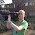2.